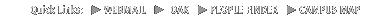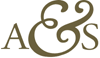John Rozen - Homepage## SOME C++ CODE...

Here are some various codes. E-mail me if you have any question or if you want a static compiled version of a program.

• C++ code used to find the conductance of a discrete network of resistors.

With this algorythm, you input a matrix of resistance values and it calculates the total conductivity of the lattice. In this version, the network has a square mesh with four resistors of equal value at each node.

This can be used to measure the total resistance of a mixture, to simulate percolation in a biphasic system... (requires cblas and clapack libraries)

Archive: percolation.zip

• C++ implementation of the Anderson mixing solving large systems of (non-)linear equations.

This method allows to solve large systems of equations having the form A*x + c*x = d or
A*x + c*x3 = d where A is a sparse matrix and c is a constant. The algorithm is very flexible and all the parameters of the mixing can be adjusted in the fucntion call.

There are three versions of this code: a serial version (for single CPU), a master/slave version and a tightly coupled version (for use on a cluster with the MPI interface). All versions require cblas and clapack libraries.